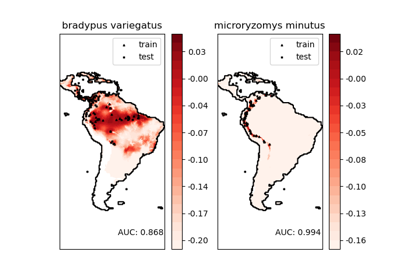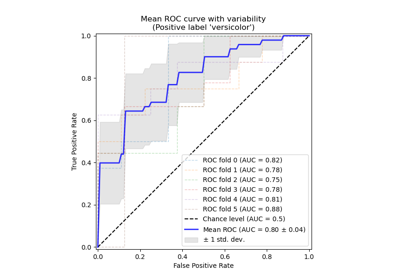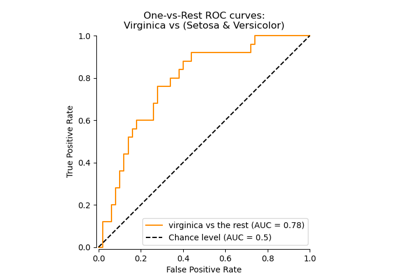# sklearn.metrics.auc¶

sklearn.metrics.auc(x, y, reorder=’deprecated’)[source]

Compute Area Under the Curve (AUC) using the trapezoidal rule

This is a general function, given points on a curve. For computing the area under the ROC-curve, see roc_auc_score. For an alternative way to summarize a precision-recall curve, see average_precision_score.

Parameters: x : array, shape = [n] x coordinates. These must be either monotonic increasing or monotonic decreasing. y : array, shape = [n] y coordinates. reorder : boolean, optional (default=’deprecated’) Whether to sort x before computing. If False, assume that x must be either monotonic increasing or monotonic decreasing. If True, y is used to break ties when sorting x. Make sure that y has a monotonic relation to x when setting reorder to True. Deprecated since version 0.20: Parameter reorder has been deprecated in version 0.20 and will be removed in 0.22. It’s introduced for roc_auc_score (not for general use) and is no longer used there. What’s more, the result from auc will be significantly influenced if x is sorted unexpectedly due to slight floating point error (See issue #9786). Future (and default) behavior is equivalent to reorder=False. auc : float

roc_auc_score
Compute the area under the ROC curve
average_precision_score
Compute average precision from prediction scores
precision_recall_curve
Compute precision-recall pairs for different probability thresholds

Examples

>>> import numpy as np
>>> from sklearn import metrics
>>> y = np.array([1, 1, 2, 2])
>>> pred = np.array([0.1, 0.4, 0.35, 0.8])
>>> fpr, tpr, thresholds = metrics.roc_curve(y, pred, pos_label=2)
>>> metrics.auc(fpr, tpr)
0.75


## Examples using sklearn.metrics.auc¶Species distribution modelingReceiver Operating Characteristic (ROC) with cross validationReceiver Operating Characteristic (ROC)